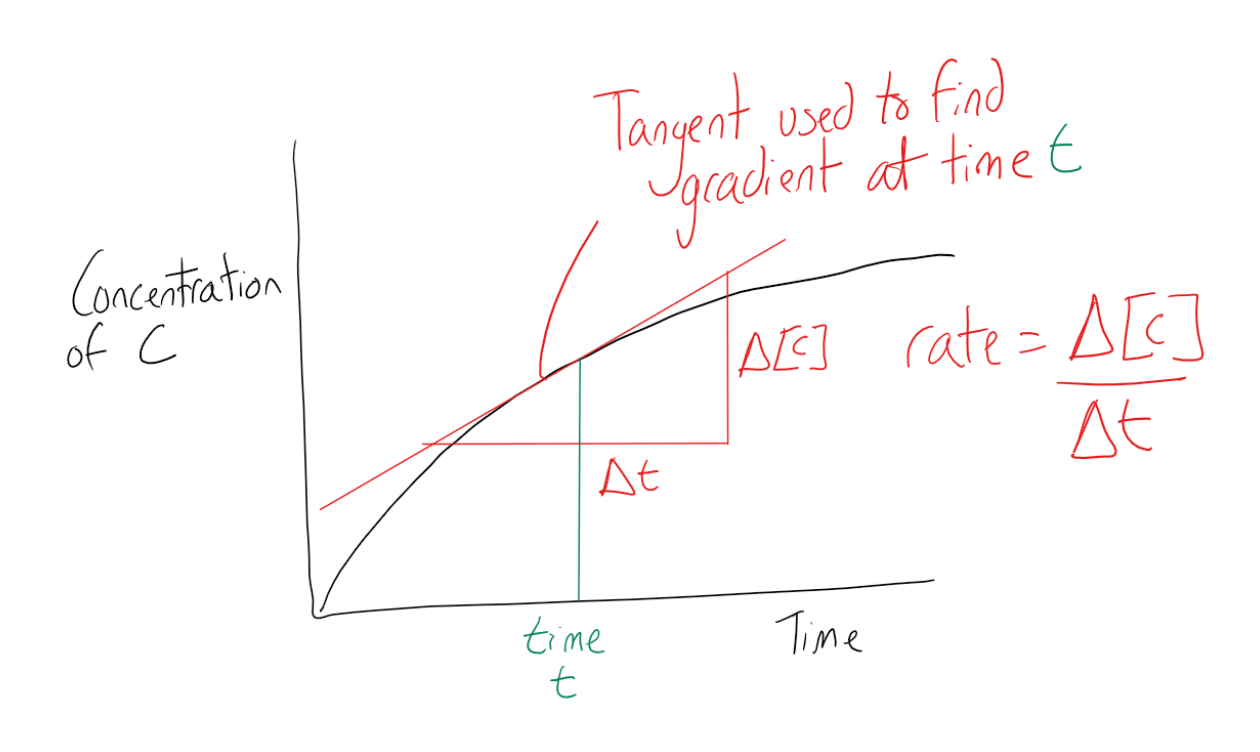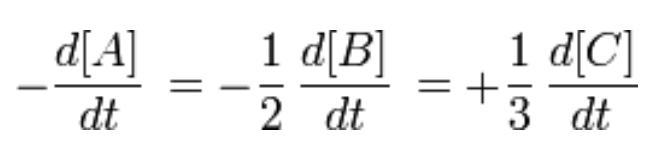Cyber Sale - Only 3 Days Remaining: ALL MCAT Products & Admissions Services

### Reaction Rate

Topic: Rate Processes In Chemical Reactions Kinetics And Equilibrium

Reaction rates are determined by observing the changes in the concentrations of reactants or products over a specific time frame.

The reaction rate is usually observed by watching the disappearance of a reactant or the appearance of a product within a given time period. Take the chemical reaction:

A+2B→3C

Here, the rate of appearance of product C in time interval Δt is:

Average rate = ΔC/Δt

The concentration of C, [C], is usually expressed in moles/liter. This is the average rate of appearance of C during the time interval Δt. The limit of this average rate as the time interval becomes smaller is called the rate of appearance of C at time t, and it is the slope of the curve of [C] versus t at time t. This instantaneous slope, or rate, is written as:

rate at time t = (d[C])/dt

The rate of reaction can be taken from a graph of time against the concentration of C where a tangent can be plotted to time and the gradient calculated to work out the rate of reaction at that exact time.The rate of change of the reactants can also be used to calculate the rate of reaction as all reactants and products are equal when considering their stoichiometric relationship. Since one molecule of A and two molecules of B are consumed for every three molecules of C that are produced, the rates of disappearance and appearance of these chemical species are different but related. This can be related by the equation below:Rates of reaction can be measured by the production of gas over time, the change in mass over time or the formation of precipitation over time.

Practice Questions

MCAT Official Prep (AAMC)

Chemistry Question Pack Passage 10 Question 59

Key Points

• The reaction rate is calculated using the formula rate = Δ[C]/Δt, where Δ[C] is the change in product concentration during time period Δt.

• The rate of reaction can be observed by watching the disappearance of a reactant or the appearance of a product over time.

• If a reaction produces a gas such as oxygen or carbon dioxide, there are two ways to measure the reaction rate: using a gas syringe to measure the gas produced or calculating the reduction in the mass of the reaction solution.

• If the reaction produces a precipitate, the amount formed can be used to determine the reaction rate by measuring how long it takes for the forming precipitate to obscure the visibility of a cross through a conical flask.

Key Terms

Reaction rate: How fast or slowly a reaction takes place.

Gas syringe: An item of laboratory equipment used to withdraw a volume of gas from a closed chemical system for measurement and/or analysis.

Product: A chemical substance formed as a result of a chemical reaction.

Billing Information